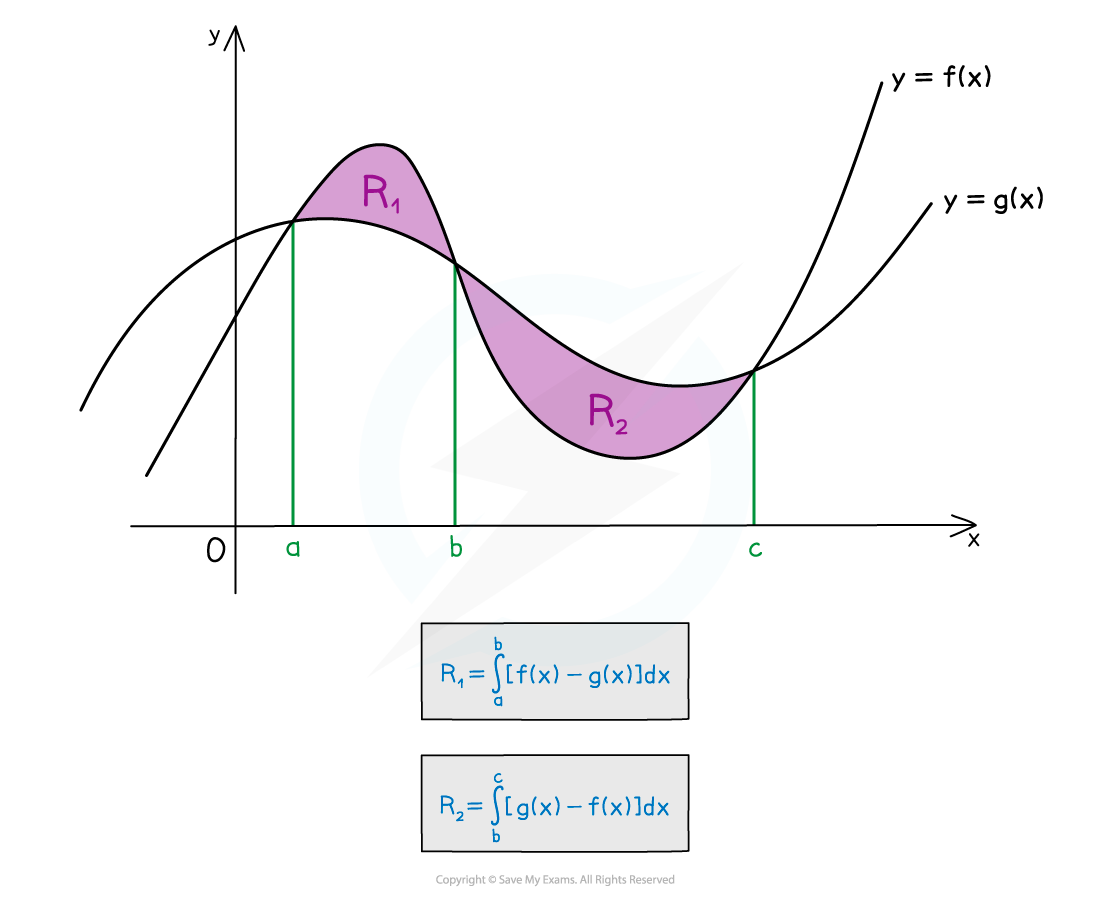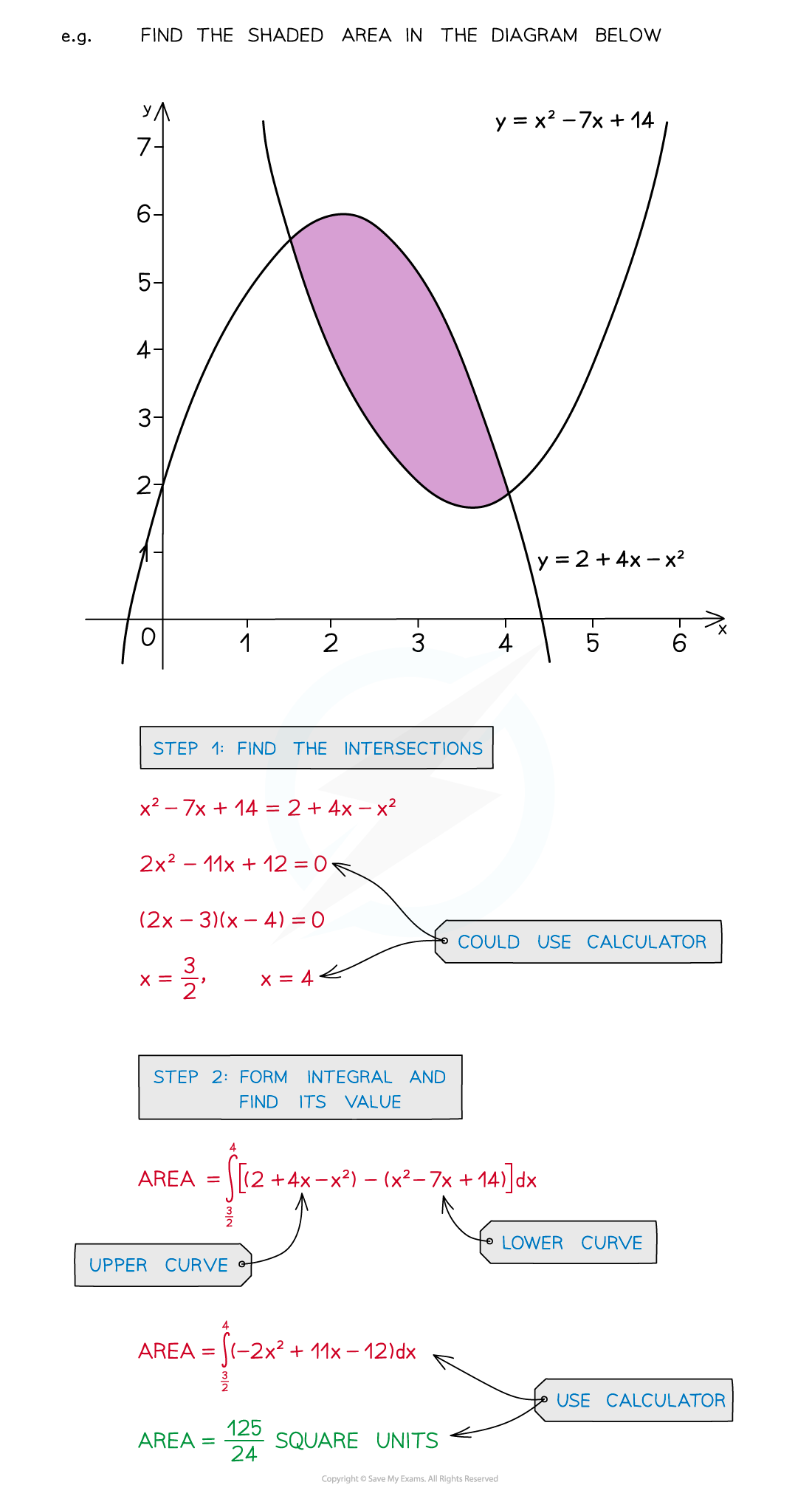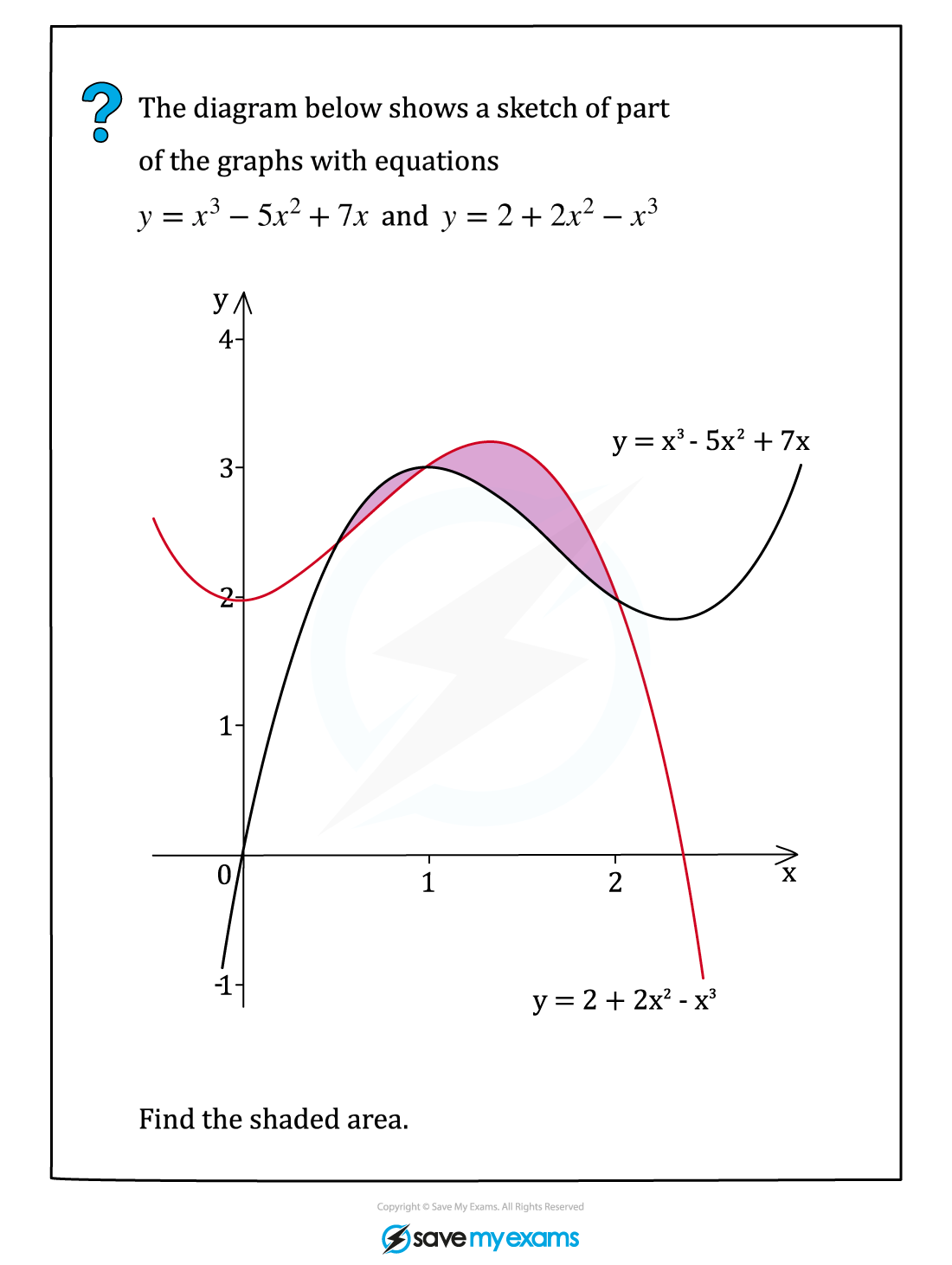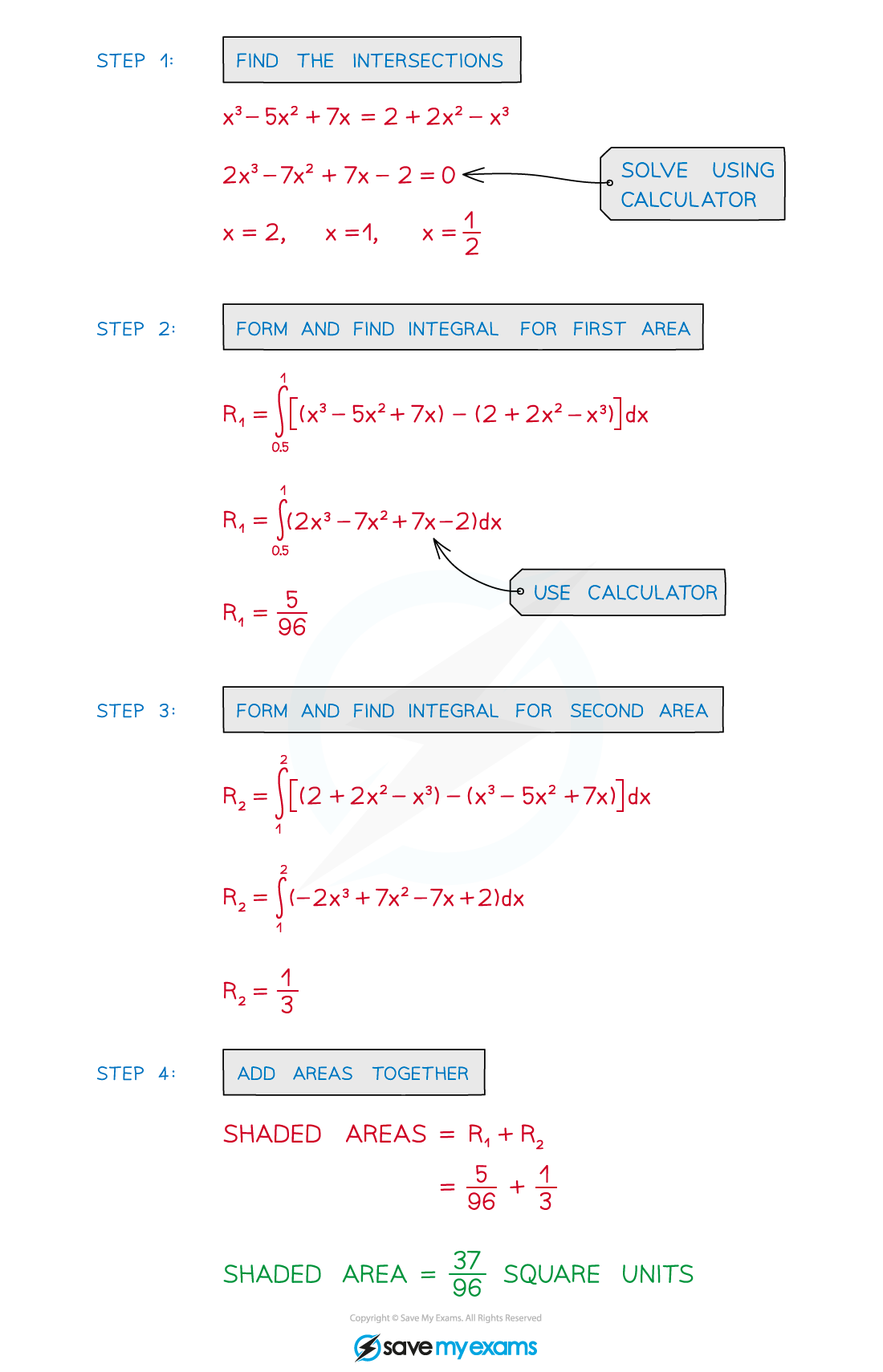# AQA A Level Maths: Pure复习笔记8.2.10 Area between 2 curves

### Area between 2 curves

#### What is the area between two curves?

• Ensure you are familiar with …
• Area under a curve
• Area between a line and a curve• In general find the definite integral of “upper curve” – “lower curve”
• However this does depend on …
• … the area being found
• … if the curves intersect (and cross over)
• The area may have to be split into separate integrals• The points at which curves intersect may need to be calculated

#### How do I find the area between two curves?•  STEP 1: Find the intersections of the curves if needed
• STEP 2: Form the integral …
• … using the intersections as limits
• … “upper curve” – “lower curve” …
• … and find the value of the integral
• STEP 3: Repeat STEP 2 if more than one area needed
• STEP 4: Add areas together

#### Exam Tip

• If no diagram is provided sketch one, even if the curves are not accurate.
• Add information to any given diagram as you work through a question.Maximise use of your calculator to save time and maintain accuracy:
• Solving equations, especially cubic equations
• Finding definite integrals

#### Worked Example